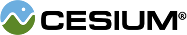#QuadraticRealPolynomial

Defines functions for 2nd order polynomial functions of one variable with only real coefficients.

### Methods

Provides the discriminant of the quadratic equation from the supplied coefficients.
Name Type Description
`a` Number The coefficient of the 2nd order monomial.
`b` Number The coefficient of the 1st order monomial.
`c` Number The coefficient of the 0th order monomial.
##### Returns:
The value of the discriminant.

`a` Number The coefficient of the 2nd order monomial.
`b` Number The coefficient of the 1st order monomial.
`c` Number The coefficient of the 0th order monomial.